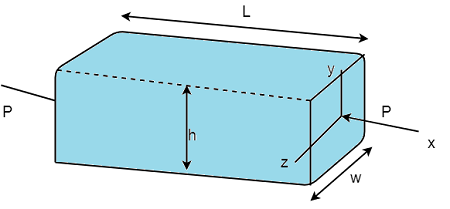# To use Hooke's law to determine the value of an axial load applied to a rectangular shape and to...

## Question:

To use Hooke's law to determine the value of an axial load applied to a rectangular shape and to determine its lateral expansion.

1). Determine the value of the axial load P.

2). Determine the lateral expansion in the y-direction due to the axial load.

3). Determine the lateral expansion in the z-direction due to the axial load.## Strain

When an amount of load act on any object then some elongation and some compression occurs in the object, the ratio of that changed dimensions in the object due to the load to the original length of the object before the applied load is known as the strain.

Become a Study.com member to unlock this answer! Create your account

Given Data

• Load acting on the block is: {eq}P {/eq}.
• Length of the block in x axis is: {eq}L {/eq}.
• Length of the block in y axis is:...